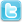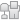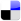ECU hacking -> Suzuki TL1000R -> Ram air maps ??
Post Info TOPIC: Ram air maps ??

Veteran Member

Status: Offline
Posts: 74
Date:
 Ram air maps ?? Permalink -- Edited by ffaspector on Saturday 17th of October 2009 11:31:03 AM __________________

Guru

Status: Offline
Posts: 964
Date:
 Permalink Actually the last map is 4 separate maps. The 96 144 16 is a map header bytes that specifies the low bound, range and interval of the X axis of the map. sub_7194 that processes these 2D maps has the value in A as an input. In this case 8 bit RPM value in 84h, Z. The routine outputs a map cell value between 0 and (range/interval) in this case 144/16 or 9 Any value 0-96 is map cell 0The high value (low+range) or 144+96 or 240 is the high bound or map cell 9The cells in between are at an interval of 16 so you get...0 = 961 = 1122 = 1283 = 1444 = 1605 = 1766 = 1927 = 2088 = 2249 = 240These values can be converted to real RPM using RPM = (value * 256 ) / 5.120 = 48001 = 56002 = 64003 = 72004 = 80005 = 88006 = 96007 = 10 4008 = 11 2009 = 12 000Also on the map values the code shows the default as 128. I would subtract 128 from your displayed map...anything under 128 being a negative offset, anything over 128 a positive value. __________________
 Page 1 of 1  sorted by Oldest FirstNewest First